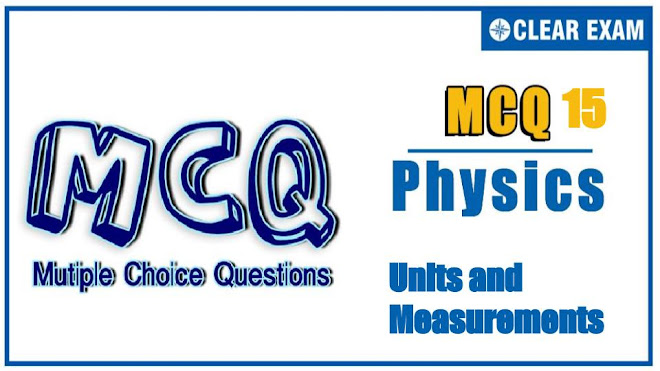## [LATEST]\$type=sticky\$show=home\$rm=0\$va=0\$count=4\$va=0

JEE Advanced paper is considered to be one of the toughest entrance exams in India. Moreover, this Physics is considered the toughest subject because it is the most application-based. The student needs to get the basics right and then move on to mastering advanced concepts. Units and Measurements consist of nearly about 3-4 % of marks in the Physics section.

Q1. Statement 1: ‘Light year’ and ‘Wavelength’ both measure distance
Statement 2: Both have dimensions of time
•  Statement 1 is True, Statement 2 is True; Statement 2 is the correct explanation for Statement 1
•  Statement 1 is True, Statement 2 is True; Statement 2 is not the correct explanation for Statement 1
•  Statement 1 is True, Statement 2 is False
•  Statement 1 is False, Statement 2 is True
Solution
(c)
Light year and wavelength both represent the distance, so both have dimensions of length not of time

Q2. Statement 1: When we change the unit of measurement of a quantity, its numerical value changes
Statement 2: Smaller the unit of measurement smaller is its numerical value
•  Statement 1 is True, Statement 2 is True; Statement 2 is the correct explanation for Statement 1
•  Statement 1 is True, Statement 2 is True; Statement 2 is not the correct explanation for Statement 1
•  Statement 1 is True, Statement 2 is False
•  Statement 1 is False, Statement 2 is True
Q3. Statement 1: Avogadro number is the number of atoms in one gram mole
Statement 2: Avogadro number is a dimensionless constant
•  Statement 1 is True, Statement 2 is True; Statement 2 is the correct explanation for Statement 1
•  Statement 1 is True, Statement 2 is True; Statement 2 is not the correct explanation for Statement 1
•  Statement 1 is True, Statement 2 is False
•  Statement 1 is False, Statement 2 is True
Solution
(c)
Avogadro number (N) represents the number of atoms in 1 gram mole of an element, i.e., it has the dimensions of mole-1

Q4. Statement 1: Light year and wavelength have same dimensions
Statement 2: Light year represents time while wavelength represents distance
•  Statement 1 is True, Statement 2 is True; Statement 2 is the correct explanation for Statement 1
•  Statement 1 is True, Statement 2 is True; Statement 2 is not the correct explanation for Statement 1
•  Statement 1 is True, Statement 2 is False
•  Statement 1 is False, Statement 2 is True
Solution
(c)
Light year and wavelength both have same dimensions of length

•  Statement 1 is True, Statement 2 is True; Statement 2 is the correct explanation for Statement 1
•  Statement 1 is True, Statement 2 is True; Statement 2 is not the correct explanation for Statement 1
•  Statement 1 is True, Statement 2 is False
•  Statement 1 is False, Statement 2 is True
Q6. The time dependence of a physical quantity P is give by P = P0 e-αt2 , where α is a constant and t is time. Then constant α is
•  Dimensionless
•  Has dimensions of T-2
•  Has dimensions of P
•  Has dimensions of T2
Q7. The dimensional formula for latent heat is
•  M0 L2 T-2
•  M L T-2
•  M L2 T-2
•  M L2 T-1
Q8. In the relation y = r sin⁡(ωt-kx), the dimensions of ω / k are
•  [M0 L0 T0]
•  [M0 L1 T-1]
•  [M0 L0 T1]
•  [M0 L1 T0]
Q9. Given that T stands for time period and l stands for the length of simple pendulum. If g is the acceleration due to gravity, then which of the following statements about the relation T2 = (l/g) is correct?
•  It is correct both dimensionally as well as numerically
•  It is neither dimensionally correct nor numerically
•  It is dimensionally correct but not numerically
•  It is numerically correct but not dimensionally
Q10. The density of a solid ball is to be determined in an experiment. The diameter of the ball is measured with a screw gauge, whose pitch is 0.5 mm and there are 50 divisions on the circular scale. The reading on the main scale is 2.5 mm and that on the circular scale is 20 divisions. If the measured mass of the ball has a relative error of 2%, the relative percentage error in the density is
•  0.9 %
•  2.4 %
•  3.1 %
•  4.2 %#### Written by: AUTHORNAME

AUTHORDESCRIPTION## Want to know more

Please fill in the details below:

## Latest NEET Articles\$type=three\$c=3\$author=hide\$comment=hide\$rm=hide\$date=hide\$snippet=hide

Name

ltr
item
BEST NEET COACHING CENTER | BEST IIT JEE COACHING INSTITUTE | BEST NEET & IIT JEE COACHING: Units and Measurements-Quiz-15
Units and Measurements-Quiz-15
https://1.bp.blogspot.com/-9J6aTWhgq8w/YORLMaUTyyI/AAAAAAAAFRc/r5yCcnG7-8wMYYz0nOQkx-pHr8ZhOvHjACLcBGAsYHQ/w660-h371/top%2Bimage%2B%252823%2529.jpg
https://1.bp.blogspot.com/-9J6aTWhgq8w/YORLMaUTyyI/AAAAAAAAFRc/r5yCcnG7-8wMYYz0nOQkx-pHr8ZhOvHjACLcBGAsYHQ/s72-w660-c-h371/top%2Bimage%2B%252823%2529.jpg
BEST NEET COACHING CENTER | BEST IIT JEE COACHING INSTITUTE | BEST NEET & IIT JEE COACHING
https://www.cleariitmedical.com/2021/07/units-and-measurements-quiz-15.html
https://www.cleariitmedical.com/
https://www.cleariitmedical.com/
https://www.cleariitmedical.com/2021/07/units-and-measurements-quiz-15.html
true
7783647550433378923
UTF-8

STAY CONNECTED